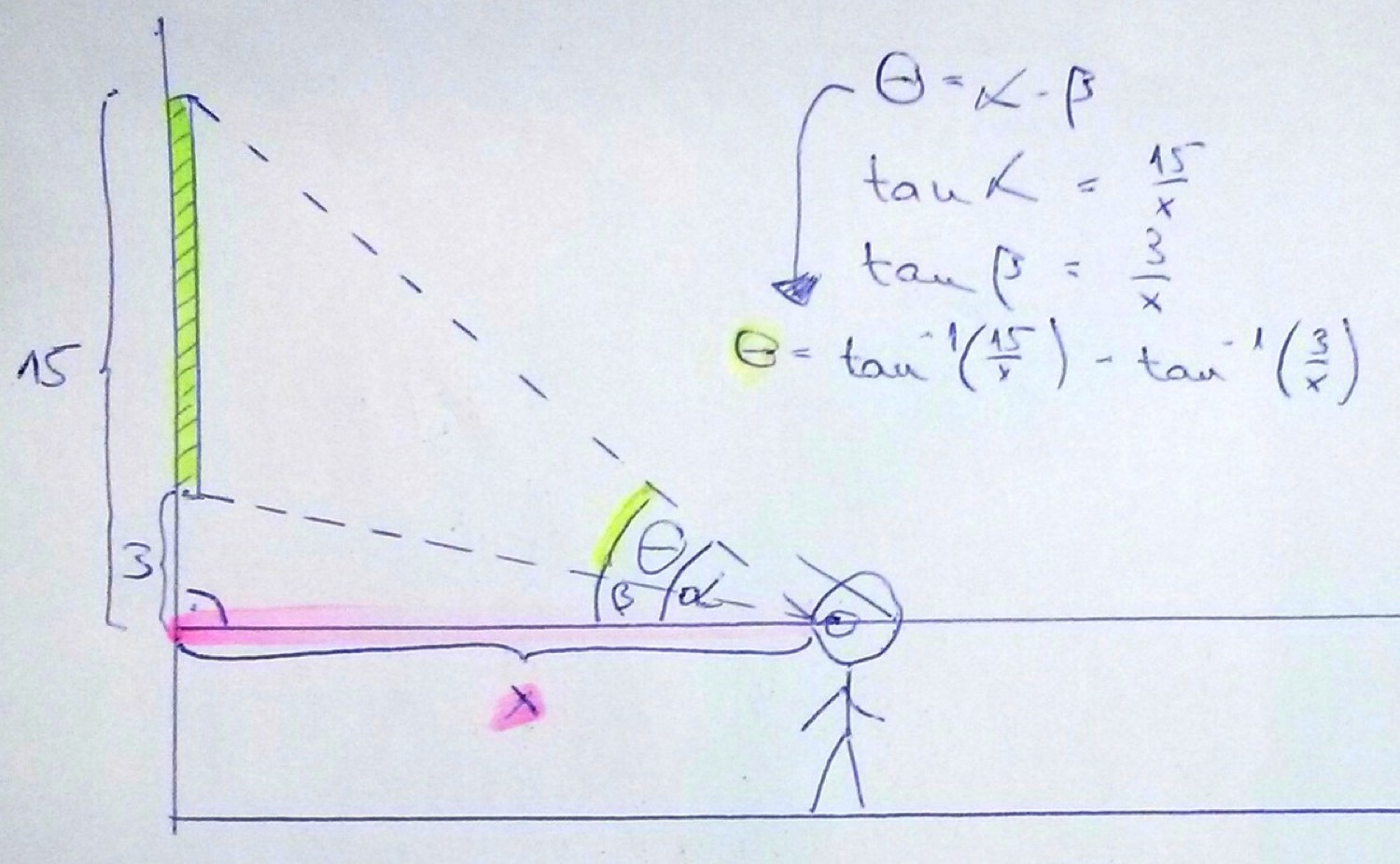## Background

SymPy is an amazing library for symbolic mathematics in Python. It’s like Mathematica, and its online shell version along with SymPy Gamma  is pretty much like Wolfram Alpha (WA).

### Problem setting

In this dummy example we’ll go to an imaginary gallery and figure out how far we should stand from a huge painting on the wall so we have the widest possible viewing angle.

If you have a look at the image below you’ll see, that the bottom of the painting is 3 meters above our level of eyesight and the top of the painting is 15 meters above it. You can imagine that if you were standing right below the painting you wouldn’t see much of it. Same thing would happen if you stood very far away. But where exactly should you stand to get the best viewing angle? I took this example from here.## Solution

So we are looking for the maximum $latex \theta$ as we are moving closer to or further away from the painting, effectively varying $latex x$. Basic geometry tells us that we can find this angle as the difference of two related angles $latex \alpha-\beta$, and trigonometry tells us that

$latex f(x) = \theta = \arctan \left(\frac{15}{x}\right)-\arctan \left(\frac{3}{x}\right)$.

### On paper

We want to find the maximum of this function with respect to $latex x$. We need to differentiate it, find its critical points and check at which of those critical points the second derivative is negative (i.e. there we’ll have the max of $latex f(x)$. We could do this manually remembering that

$latex \frac{d}{dx}\arctan x=\frac{1}{1+x^2},$

but what’s the fun in that? :)

### Using SymPy

So let’s type a few lines of code into SymPy’s online shell:

### Breaking it down

1. In the first line we define $latex f(x)$.
• The cool thing here is that this is done symbolically, as we would do it on a piece of paper.
2. In the 2nd line we differentiate this function.
• This is done as it would be done on paper. If you now type $latex df$, you’ll get the derivative:
• $latex f’(x) = -\frac{15}{x^2 \left( 1+\frac{255}{x^2}\right)}+\frac{3}{x^2 \left( 1+\frac{9}{x^2}\right)}$
• Even if I had looked up $latex \frac{d}{dx} \text{arctan}(x)$, it would have taken a bit of time for me to get here to be honest..
3. In the third line we get the second derivative,
4. In the 4th we find the critical points of $latex f(x)$.
• Again everything is symbolic, nothing would make sense in pure Python, but it works beautifully in SymPy.
5. In the 5th line we print the critical points.
• We get $latex -3\sqrt{5}$ and $latex 3\sqrt{5}$.
• Remember, we are looking for $latex x$, which is a distance, so we should be suspicious(to say the least) about the negative value, but we still need to check that $latex f(x)$ actually has a local maximum at $latex 3\sqrt{5}$.
6. That’s exactly what the 6th line does.
• We substitute in the second critical point into the second derivative, and indeed we get a negative value, confirming that $latex f(x)$ has a local maximum at $latex 3\sqrt{5}$.
7. In the 7th line we use the $latex N()$ function to print out the numerical value of this expression, which is $latex \approx$ 6.7 meters.

## Summary

So we should stand about 6.7 meters away from the painting to get the maximum possible viewing angle.

There you go, in less than 10 lines we did some optimization symbolically in Python. Actually we could have done this in about three lines, sacrificing a bit of clarity, but that’s never a good trade-off..

As I said, this is barely scratching the surface of what SymPy can do for you, but if you were after an alternative of Wolfram Alpha for symbolic mathematics, I think you’ll find it extremely helpful.

Tags:

Topics:

Updated: